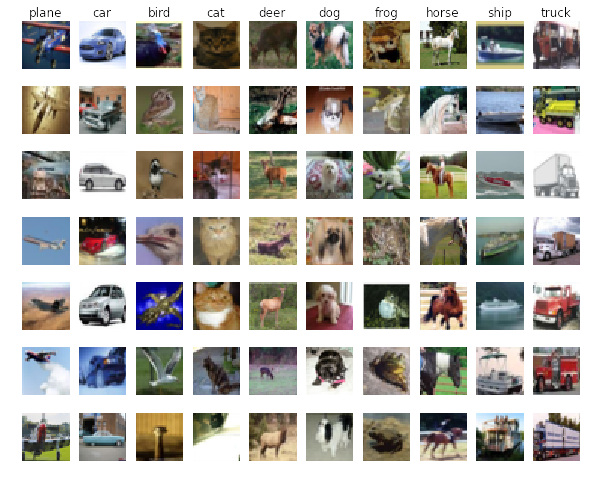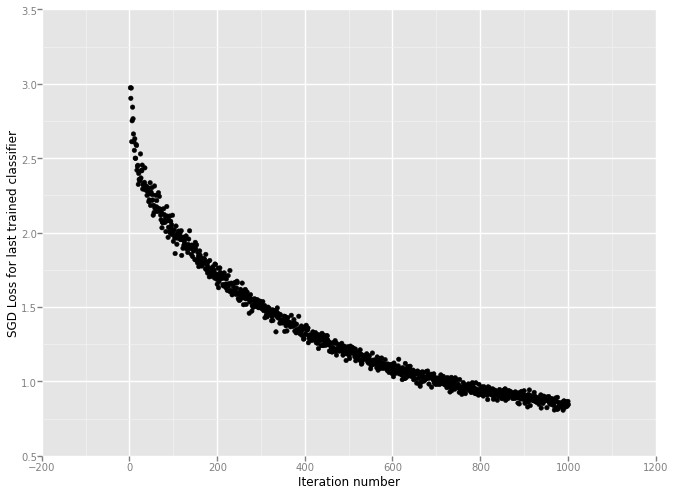## Multi-classification based One-vs-All Logistic Regression

### Building one-vs-all logistic regression classifiers to distinguish ten objects in CIFAR-10 dataset, the binary logistic classifier implementation is here.

Most of the codes are copied from binary logistic implementation to make this notebook self-contained.

• implement a fully-vectorized loss function for the Logistic Regression
• implement the fully-vectorized expression for its analytic gradient
• check implementation using numerical gradient
• optimize the loss function with Batch Gradient Descent and Stochastic Gradient Descent
In :
# Setup code for this notebook
import random
import numpy as np
import matplotlib.pyplot as plt

# This is a bit of magic gto make matplotlib figures appear inline
# in the notebook rather than in a new window
%matplotlib inline
plt.rcParams['figure.figsize'] = (10.0, 8.0)
plt.rcParams['image.interpolation'] = 'nearest'
plt.rcParams['image.cmap'] = 'gray'
# Some more magic so that the notebook will reload external python modules;


get_datasets.sh

# Get CIFAR10
wget http://www.cs.toronto.edu/~kriz/cifar-10-python.tar.gz
tar -xzvf cifar-10-python.tar.gz
rm cifar-10-python.tar.gz## Write function to load data in data_utils.py

In :
# Write function to load the cifar-10 data
# The original code is from http://cs231n.github.io/assignment1/
# The function is in data_utils.py file for reusing.
import cPickle as pickle
import numpy as np
import os

""" load single batch of cifar """
with open(filename, 'r') as f:
X = X.reshape(10000, 3, 32, 32).transpose(0,2,3,1).astype("float")
Y = np.array(Y)
return X, Y

""" load all of cifar """
xs = []
ys = []
for b in range(1,6):
f = os.path.join(ROOT, 'data_batch_%d' % (b, ))
xs.append(X)
ys.append(Y)
Xtr = np.concatenate(xs)
Ytr = np.concatenate(ys)
del X, Y
return Xtr, Ytr, Xte, Yte


## Load data and visualize some samples

In :
from algorithms.data_utils import load_CIFAR10
classes = ['plane', 'car', 'bird', 'cat', 'deer', 'dog', 'frog', 'horse', 'ship', 'truck']

def get_CIFAR10_data(num_training=49000, num_val=1000, num_test=10000, show_sample=True):
"""
Load the CIFAR-10 dataset, and divide the sample into training set, validation set and test set
"""

cifar10_dir = 'datasets/datasets-cifar-10/cifar-10-batches-py/'
X_train, y_train, X_test, y_test = load_CIFAR10(cifar10_dir)

# subsample the data for validation set
mask = xrange(num_training, num_training + num_val)
return X_train, y_train, X_val, y_val, X_test, y_test

def visualize_sample(X_train, y_train, classes, samples_per_class=7):
"""visualize some samples in the training datasets """
num_classes = len(classes)
for y, cls in enumerate(classes):
idxs = np.flatnonzero(y_train == y) # get all the indexes of cls
idxs = np.random.choice(idxs, samples_per_class, replace=False)
for i, idx in enumerate(idxs): # plot the image one by one
plt_idx = i * num_classes + y + 1 # i*num_classes and y+1 determine the row and column respectively
plt.subplot(samples_per_class, num_classes, plt_idx)
plt.imshow(X_train[idx].astype('uint8'))
plt.axis('off')
if i == 0:
plt.title(cls)
plt.show()

def preprocessing_CIFAR10_data(X_train, y_train, X_val, y_val, X_test, y_test):

# Preprocessing: reshape the image data into rows
X_train = np.reshape(X_train, (X_train.shape, -1)) # [49000, 3072]
X_val = np.reshape(X_val, (X_val.shape, -1)) # [1000, 3072]
X_test = np.reshape(X_test, (X_test.shape, -1)) # [10000, 3072]

# Normalize the data: subtract the mean image
mean_image = np.mean(X_train, axis = 0)
X_train -= mean_image
X_val -= mean_image
X_test -= mean_image

# Add bias dimension and transform into columns
X_train = np.hstack([X_train, np.ones((X_train.shape, 1))]).T
X_val = np.hstack([X_val, np.ones((X_val.shape, 1))]).T
X_test = np.hstack([X_test, np.ones((X_test.shape, 1))]).T
return X_train, y_train, X_val, y_val, X_test, y_test

# Invoke the above functions to get our data
X_train_raw, y_train_raw, X_val_raw, y_val_raw, X_test_raw, y_test_raw = get_CIFAR10_data()
visualize_sample(X_train_raw, y_train_raw, classes)

X_train, y_train, X_val, y_val, X_test, y_test = preprocessing_CIFAR10_data(X_train_raw, y_train_raw, X_val_raw, y_val_raw, X_test_raw, y_test_raw)

# As a sanity check, we print out th size of the training and test data dimenstion
print 'Train data shape: ', X_train.shape
print 'Train labels shape: ', y_train.shape
print 'Validation data shape: ', X_val.shape
print 'Validation labels shape: ', y_val.shape
print 'Test data shape: ', X_test.shape
print 'Test labels shape: ', y_test.shapeTrain data shape:  (3073, 49000)
Train labels shape:  (49000,)
Validation data shape:  (3073, 1000)
Validation labels shape:  (1000,)
Test data shape:  (3073, 10000)
Test labels shape:  (10000,)



## One-vs-All Logisic Regression Classifier for Multi-classification

The code is running in the backend, you can find it here, or github

The basic idea is to change multiple classes into two classes, and construct one logistic classifier for each class. We set the value of y (label) of one class to 1, and 0 for other classes.

## Firstly test the gradient and loss function

In :
# Set the label of the first class to be one, and 0 for others
from copy import deepcopy
y_train_test_loss = deepcopy(y_train)
idxs_zero = y_train_test_loss == 0
y_train_test_loss[idxs_zero] = 1
y_train_test_loss[-idxs_zero] = 0

# Test the loss and gradient and compare between two implementations
import time

# generate a rand weights W
W = np.random.randn(1, X_train.shape) * 0.001
tic = time.time()
toc = time.time()
print 'Naive loss: %f, and gradient: computed in %fs' % (loss_naive, toc - tic)

tic = time.time()
toc = time.time()
print 'Vectorized loss: %f, and gradient: computed in %fs' % (loss_vec, toc - tic)

# Compare the gradient, because the gradient is a vector, we canuse the Frobenius norm to compare them
# the Frobenius norm of two matrices is the square root of the squared sum of differences of all elements
# Randomly choose some gradient to check
idxs = np.random.choice(X_train.shape, 10, replace=False)
print idxs
print 'Gradient difference between naive and vectorized version is: %f' % diff

Naive loss: 1.221357, and gradient: computed in 60.720513s
Vectorized loss: 1.221357, and gradient: computed in 0.130304s
[1124 1374 2316 1795 2360  578 2490  883 2823 1384]
[ 1.61441473  1.468119   -6.47071509 -1.13203816 -1.48937488  0.41119585
-4.99396434  4.1517574  -5.67236155  3.32974893]
[ 1.61441473  1.468119   -6.47071509 -1.13203816 -1.48937488  0.41119585
-4.99396434  4.1517574  -5.67236155  3.32974893]
Gradient difference between naive and vectorized version is: 0.000000



## Write function to compute gradient numerically for test the analytic gradient

And put the function in algorithms/gradient_check.py file for future use

In :
# file: algorithms/gradient_check.py
"""
sample a few random elements and only return numerical
in this dimensions.
"""
h = 1e-5

print x.shape

for i in xrange(num_checks):
ix = tuple([randrange(m) for m in x.shape])
print ix
x[ix] += h # increment by h
fxph = f(x) # evaluate f(x + h)
x[ix] -= 2 * h # increment by h
fxmh = f(x) # evaluate f(x - h)
x[ix] += h # reset

grad_numerical = (fxph - fxmh) / (2 * h)
print 'numerical: %f analytic: %f, relative error: %e' % (grad_numerical, grad_analytic, rel_error)

f = lambda w: loss_grad_logistic_vectorized(w, X_train, y_train, 0)

(1, 3073)
(0, 1812)
numerical: 5.611695 analytic: -1.265780, relative error: 1.000000e+00
(0, 2960)
numerical: 13.760122 analytic: -4.213841, relative error: 1.000000e+00
(0, 1290)
numerical: -3.100102 analytic: 4.186288, relative error: 1.000000e+00
(0, 2398)
numerical: 7.901516 analytic: -5.015098, relative error: 1.000000e+00
(0, 2418)
numerical: 8.636010 analytic: -5.322674, relative error: 1.000000e+00
(0, 307)
numerical: -7.359046 analytic: 1.170376, relative error: 1.000000e+00
(0, 2052)
numerical: 2.100902 analytic: -0.113205, relative error: 1.000000e+00
(0, 1408)
numerical: 6.826968 analytic: 0.201099, relative error: 9.427725e-01
(0, 2831)
numerical: 17.535111 analytic: -4.311547, relative error: 1.000000e+00
(0, 1493)
numerical: 11.041753 analytic: 4.239572, relative error: 4.451303e-01



## Set up and train 10 logistic classifier

In :
# train 10 logistic classifier
from algorithms.classifiers import Logistic

logistic_classifiers = []
num_classes = np.max(y_train) + 1
losses = []
for i in xrange(num_classes):
print '\nThe %d/%dth logistic classifier training...' % (i+1, num_classes)
y_train_logistic = deepcopy(y_train)
idxs_i = y_train_logistic == i
y_train_logistic[idxs_i] = 1
y_train_logistic[-idxs_i] = 0
logistic = Logistic()
loss = logistic.train(X_train, y_train_logistic, method='sgd', batch_size=200, learning_rate=1e-6,
reg = 1e3, num_iters=1000, verbose=True, vectorized=True)
losses.append(loss)
logistic_classifiers.append(logistic)


The 1/10th logistic classifier training...
iteration 0/1000: loss 3.084220
iteration 100/1000: loss 2.095845
iteration 200/1000: loss 1.704919
iteration 300/1000: loss 1.497091
iteration 400/1000: loss 1.358109
iteration 500/1000: loss 1.223217
iteration 600/1000: loss 1.092730
iteration 700/1000: loss 1.040516
iteration 800/1000: loss 0.934460
iteration 900/1000: loss 0.900970

The 2/10th logistic classifier training...
iteration 0/1000: loss 3.486220
iteration 100/1000: loss 1.969887
iteration 200/1000: loss 1.716406
iteration 300/1000: loss 1.516896
iteration 400/1000: loss 1.324637
iteration 500/1000: loss 1.180693
iteration 600/1000: loss 1.101289
iteration 700/1000: loss 1.008053
iteration 800/1000: loss 0.912884
iteration 900/1000: loss 0.906440

The 3/10th logistic classifier training...
iteration 0/1000: loss 2.854838
iteration 100/1000: loss 1.885625
iteration 200/1000: loss 1.710971
iteration 300/1000: loss 1.506277
iteration 400/1000: loss 1.346721
iteration 500/1000: loss 1.216073
iteration 600/1000: loss 1.097695
iteration 700/1000: loss 1.020052
iteration 800/1000: loss 0.954086
iteration 900/1000: loss 0.906712

The 4/10th logistic classifier training...
iteration 0/1000: loss 3.029458
iteration 100/1000: loss 2.026928
iteration 200/1000: loss 1.827198
iteration 300/1000: loss 1.522781
iteration 400/1000: loss 1.355208
iteration 500/1000: loss 1.240110
iteration 600/1000: loss 1.143533
iteration 700/1000: loss 1.067226
iteration 800/1000: loss 0.975935
iteration 900/1000: loss 0.902043

The 5/10th logistic classifier training...
iteration 0/1000: loss 2.928803
iteration 100/1000: loss 2.009902
iteration 200/1000: loss 1.787873
iteration 300/1000: loss 1.496266
iteration 400/1000: loss 1.386474
iteration 500/1000: loss 1.218843
iteration 600/1000: loss 1.134146
iteration 700/1000: loss 1.069044
iteration 800/1000: loss 0.940430
iteration 900/1000: loss 0.936724

The 6/10th logistic classifier training...
iteration 0/1000: loss 2.809468
iteration 100/1000: loss 2.104138
iteration 200/1000: loss 1.774723
iteration 300/1000: loss 1.506205
iteration 400/1000: loss 1.358423
iteration 500/1000: loss 1.239632
iteration 600/1000: loss 1.093584
iteration 700/1000: loss 1.003983
iteration 800/1000: loss 0.975950
iteration 900/1000: loss 0.904384

The 7/10th logistic classifier training...
iteration 0/1000: loss 2.949246
iteration 100/1000: loss 2.046533
iteration 200/1000: loss 1.763477
iteration 300/1000: loss 1.519313
iteration 400/1000: loss 1.348424
iteration 500/1000: loss 1.131125
iteration 600/1000: loss 1.145246
iteration 700/1000: loss 1.045521
iteration 800/1000: loss 0.977439
iteration 900/1000: loss 0.911603

The 8/10th logistic classifier training...
iteration 0/1000: loss 2.998891
iteration 100/1000: loss 2.056269
iteration 200/1000: loss 1.740083
iteration 300/1000: loss 1.489378
iteration 400/1000: loss 1.264001
iteration 500/1000: loss 1.221685
iteration 600/1000: loss 1.129864
iteration 700/1000: loss 1.033490
iteration 800/1000: loss 0.946090
iteration 900/1000: loss 0.931818

The 9/10th logistic classifier training...
iteration 0/1000: loss 3.282589
iteration 100/1000: loss 2.089450
iteration 200/1000: loss 1.818178
iteration 300/1000: loss 1.496629
iteration 400/1000: loss 1.394318
iteration 500/1000: loss 1.179894
iteration 600/1000: loss 1.114139
iteration 700/1000: loss 0.989329
iteration 800/1000: loss 0.925881
iteration 900/1000: loss 0.894325

The 10/10th logistic classifier training...
iteration 0/1000: loss 2.979882
iteration 100/1000: loss 2.024313
iteration 200/1000: loss 1.767455
iteration 300/1000: loss 1.495541
iteration 400/1000: loss 1.321874
iteration 500/1000: loss 1.160301
iteration 600/1000: loss 1.038245
iteration 700/1000: loss 1.001797
iteration 800/1000: loss 0.924224
iteration 900/1000: loss 0.859794


In :
from ggplot import *
# plot the loss of the last trained logistic classifier
qplot(xrange(len(losses)), losses) + labs(x='Iteration number', y='SGD Loss for last trained classifier')Out:
<ggplot: (402325625)>


## Prediction by using trained 10 logistic classifiers

Choose the class with the largest score

In :
# Compute the accuracy of training data and validation data
def predict_one_vs_all(logistic_classifiers, X, num_classes):
scores = np.zeros((num_classes, X.shape))
for i in xrange(num_classes):
logistic = logistic_classifiers[i]
scores[i, :] = logistic.predict(X)
pred_X = np.argmax(scores, axis=0)
return pred_X

pred_train_one_vs_all = predict_one_vs_all(logistic_classifiers, X_train, num_classes)
pred_val_one_vs_all = predict_one_vs_all(logistic_classifiers, X_val, num_classes)
pred_test_one_vs_all = predict_one_vs_all(logistic_classifiers, X_test, num_classes)
print 'Training dataset accuracy: %f' % (np.mean(y_train == pred_train_one_vs_all))
print 'Validation dataset accuracy: %f' % (np.mean(y_val == pred_val_one_vs_all))
print 'Test datast accuracy: %f' % (np.mean(y_test == pred_test_one_vs_all))

Training dataset accuracy: 0.350531
Validation dataset accuracy: 0.335000
Test datast accuracy: 0.334600



## A little summary

• The accuracy for test dataset can reach 33.70%, which is acceptable when compared with randomly chosing (10.00%)
• The performance could be improved to tune learning rate and regularization strength by validation datasets
• Noctice that we don't directly use the final prediction of logistic regression classifier, instead using the scores for multi-classification. Because there is a potential problem that one sample might be classified to several classes or non-class by fully using logistic regression prediction
In :